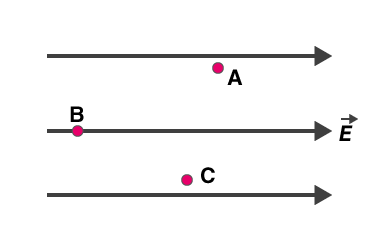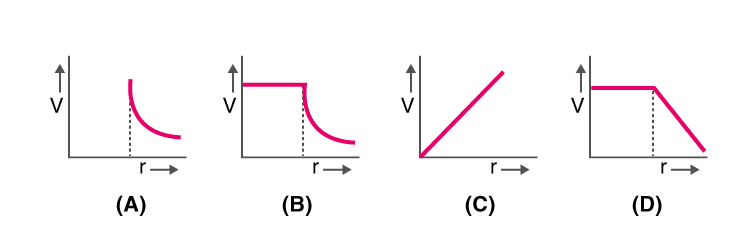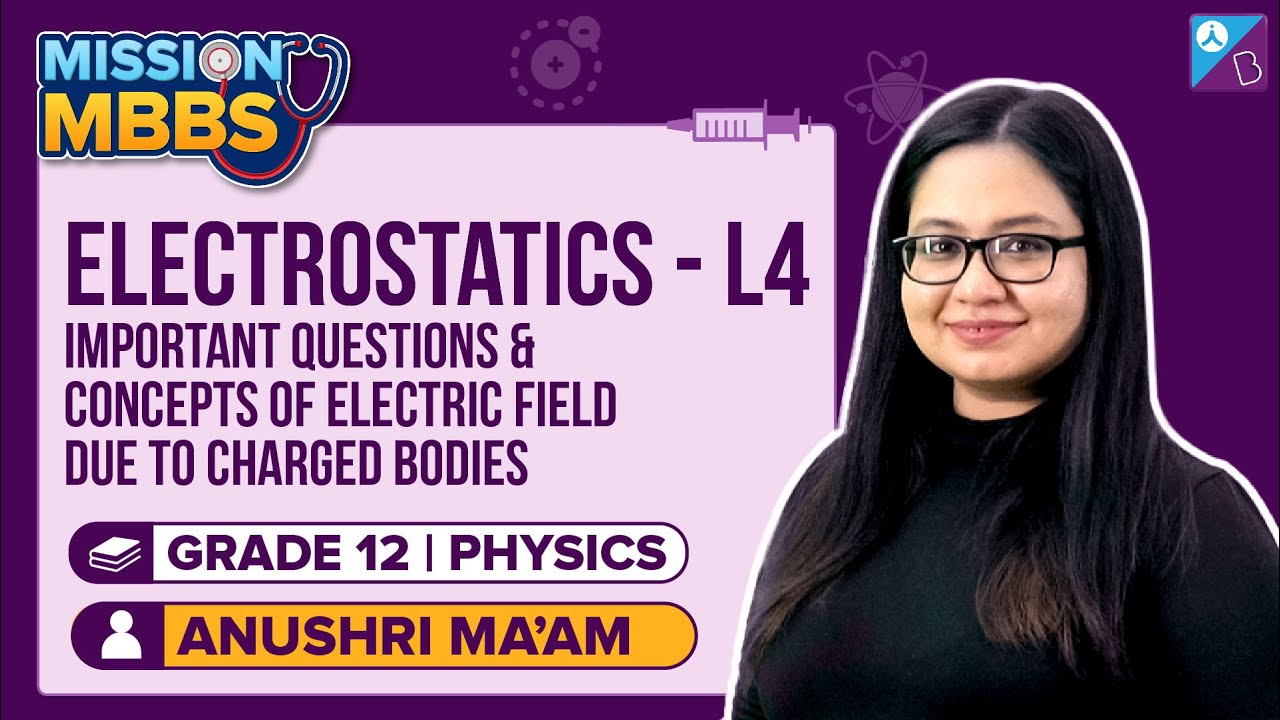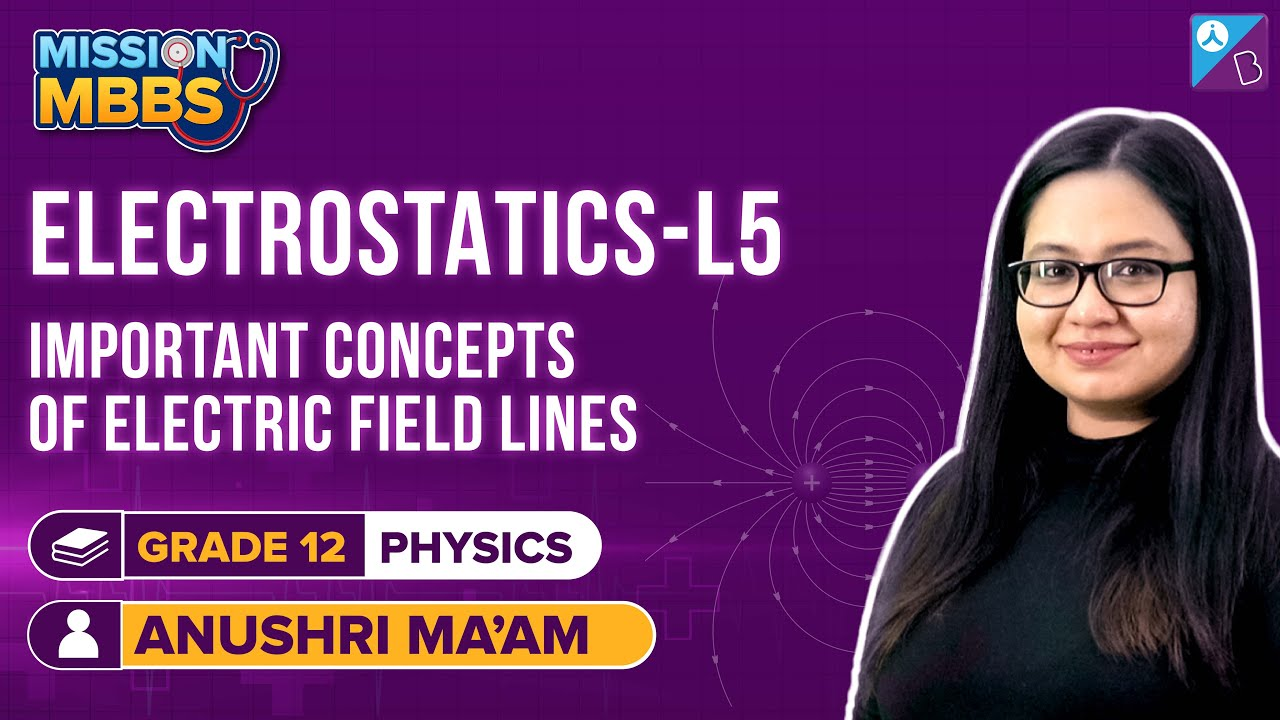Win up to 100% scholarship on Aakash BYJU'S JEE/NEET courses with ABNAT Win up to 100% scholarship on Aakash BYJU'S JEE/NEET courses with ABNAT

# Electro statistics MCQs for NEET

Electrostatics is the study of stationary electric charges. An example of electrostatics is when a plastic rod is rubbed with the fur or silk, it will start to attract tiny pieces of paper and the rod is said to be electrically charged. The charge acquired by the plastic rod is negative and the paper is positive.

Following are the characteristics of electric charge:

• Like charges repel each other while unlike charges attract each other.
• A charge is conserved.
• All the charges are governed by Coulomb’s law.

1. What will be the flux coming out of any surface a cube, if a change QμC is placed at the centre of the cube?

$$\begin{array}{l}(a) \frac{Q}{6\epsilon _{0}}\times 10^{-3}\end{array}$$
$$\begin{array}{l}(b) \frac{Q}{24\epsilon _{0}}\end{array}$$
$$\begin{array}{l}(c) \frac{Q}{8\epsilon _{0}}\end{array}$$
$$\begin{array}{l}(d) \frac{Q}{6\epsilon _{0}}\times 10^{-6}\end{array}$$
$$\begin{array}{l}Answer. (d) \frac{Q}{6\epsilon _{0}}\times 10^{-6}\end{array}$$

2. Name the law that states the force between electric charges

(a) Ohm’s law

(b) Coulomb’s law

(d) Ampere’s law

3. What happens to the force acting between the charged particles, if the distance between these charged particles is halved?

(a) It increases by four times

(b) It gets doubled

(c) It becomes half

(d) It reduces by one-fourth

Answer: (a) It increases by four times

4. What is the electric potential of the points A, B, and C which are in a uniform electric field?(a) The electric field is the same at all three points A, B, and C
(b) Maximum at A
(c) Maximum at B
(d) Maximum at C

5. What is the minimum charge on a particle?
(a) 1 Coulomb
(b) 1.6 x 10-19 Coulomb
(c) 3.2 x 10-19 Coulomb
(d) 6.6 x 10-19 Coulomb
Answer: (b) 1.6 x 10-19 Coulomb

6. For a hollow spherical shell, potential, V changes with respect to distance, r from the centre. Which graph from the following represents this?7. What is the graphical representation of an electric field due to the uniformly charged sphere of radius R which is a function of distance from the centre?8. The capacity of parallel plate condenser is dependent on the

(a) The separation between the plates

(b) The metal used for the construction

(c) The thickness of the plate

(d) The potential applied across the plates

Answer: (a) The separation between the plates

9. The mathematical representation of the force of attraction between the plates of the parallel plate capacitor is given as

$$\begin{array}{l}(a) \frac{q^{2}}{2\epsilon _{0}A^{2}K}\end{array}$$
$$\begin{array}{l}(b) \frac{q^{2}}{\epsilon _{0}AK}\end{array}$$
$$\begin{array}{l}(c)\frac{q^{2}}{2\epsilon _{0}AK}\end{array}$$
$$\begin{array}{l}(d) \frac{q}{2\epsilon _{0}A}\end{array}$$
$$\begin{array}{l}Answer. \ (c)\frac{q^{2}}{2\epsilon _{0}AK}\end{array}$$

10. What does an electric dipole experience when it is kept in the non-uniform electric field?

(a) Only a force

(b) Only torque

(c) Force and torque both

(d) Neither force nor torque

Answer: (c) Force and torque both

11. The capacitance of the capacitor is independent of

(a) The charges present on the plate

(b) The distance of separation between the plates

(c) The shape of the plates

(d) The size of the plates

Answer: (d) The size of the plates

12. Consider two capacitances of capacity C1 and C2 which are connected in series and have potential difference V. What is the potential difference across C1?

$$\begin{array}{l}(a) V(\frac{C_{1}}{C_{1}+C_{2}})\end{array}$$
$$\begin{array}{l}(b) V(\frac{C_{1}+C_{2}}{C_{1}})\end{array}$$
$$\begin{array}{l}(c) V(\frac{C_{2}}{C_{1}})\end{array}$$
$$\begin{array}{l}(d) V(\frac{C_{2}}{C_{1}+C_{2}})\end{array}$$
$$\begin{array}{l}Answer. (d) V(\frac{C_{2}}{C_{1}+C_{2}})\end{array}$$